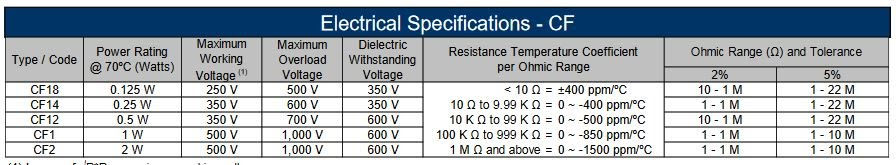top of page
Search

# UNDERSTANDING POWER AND HEATSINKS PART I - THEORY

Updated: Nov 16, 2020

Heat in electronics is inevitable but improper management of heat can be catastrophic. Learn about how manage heat in your next project.In electronics, power usually refers to heat generated by a component. There are exceptions of course, like the power generated by an electric motor. Power in this case represents the mechanical power generated from the electrical energy. However in this article, we will be talking about power generated as heat in a component.

All components have a power rating. Resistors for example, have a direct power rating. ie. 1/8W, 1/4W and so on. Semiconductors that can be attached to a heatsink on the other hand are typically rated in thermal resistance. ie. Rja, Rjc etc

Example of Resistor Power RatingExample of TO220-3 Package Thermal Specs.A component left in free air uses the Junction to Ambient characteristic (Rja). This is the thermal resistance from the semiconductor die inside the component to the air. Whereas a component with a heat sink uses to total thermal resistances of all the parts involved. In this case the semiconductor uses the Junction to Case resistance(Rjc). This is the thermal resistance from the semiconductor die to the case of the component and is quite a bit lower than the Junction to Ambient.

The units used for thermal resistance are °C/W, in other words, for every watt of heat the component dissipates, it raises the junction temperature by the specified value. For example, refer to this schematic.In this case the voltage across the regulator is 7V (12V source - 5V load), the load current is 0.5A (I=V/R or 5V/10ohms = 0.5A). So the power dissipated by the regulator is

3.5W (P=V*I or 7V * 0.5A = 3.5W).

Knowing that our Rja = 23.9°C/W from the chart above,

the temperature rise = 3.5W * 23.9°C/W = 83.65°C above ambient. So the final junction temperature will be 83.65°C + Room Temp(21°C) = 104.65°C

The next important characteristic we need is the maximum junction temperature. This temperature is the value that the component can reach without damage. Anything above this can result in failure of the part. However, it's not good practice to run a part near this temperature for long periods of time as it will likely increase the odds of the part failing. Note the maximum junction temperature of our regulator below.Here our max temp is 150°C and our current design will be well below the max and although hot, will run without too much trouble. In addition, there's a recommended operating temperature and for this regulator that value is 125°C and is the temperature at which should not be exceed under normal operating conditions.

What would happen if we needed the full 1A available to us from the regulator? Using the above method, we would calculate the junction temperature as follows.

Power = V * I = 7V * 1A = 7W     Trise = Rja * P = 23.9°C/W * 7W = 167.3°C     Tj = Trise + Tamb = 167.3° + 21° = 188.3°C

This would result in a temperature exceeding the max junction temp and causing a thermal shutdown of the part in this case. Some parts have no thermal shutdown and this would result in seeing the magic blue smoke. To use the full capacity of the regulator, we need a way of removing the heat from the part. This is where a heat sink comes into play.

Heat sinks all have thermal resistance ratings and the lower the value, the more heat it is able to dissipate. In addition, the lower the thermal resistance, the larger and bulkier the heat sink. Examine the 4 heat sinks below.Using a heat sink requires the addition of all the thermal resistances in the path the heat will take. So for example, if we use the 12°C/W heat sink and a thermal pad with a thermal resistance of 0.35°C/W, our total thermal resistance is

Tja = Regulator Rjc + heat sink resistance + thermal pad resistance

= 1.7°C/W + 12°C/W + 0.35°C/W = 14.05°C/WWith this new Junction to ambient value we can re-evaluate our case from above.     Power is still 7W     The new Trise = 14.05°C/W * 7W = 98.35°C     Tj = 98.35° + 21° = 119.35°

The final temperature now being well below the max junction temperature and just below the maximum operating temperature, we can now draw 1A continuously. One last point to mention is what is the maximum ambient temperature that can be used? This is calculated by subtracting the maximum temperature rise from the maximum junction temperature.

It should be noted that there can be a large difference between the theoretical and practical ratings as we shall see in part II.

From our example above, Maximum ambient temp = 150° - 98.35° = 51.65°C So at full load, the regulator will function to a maximum of about 52°C ambient temperature.     In Part II of this article, I will demonstrate some real world examples using a 5V regulator and measuring the temperature change over time with a given load on 4 different heat sink types.